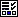#MathCS.org - Statistics

## Introduction to Statistics with Excel

 This section includes a complete introductory course on statistical analysis, using Excel as a (sophisticated) "stats calculator". No prior experience with Excel or Statistics is required, the course is completely self-contained. Have fun, and may all your statistics be significant ...

### 1. Introduction1. Overview
2. What is Statistics
3. What is a "Random Sample"
4. Variables and distributions
5.Quiz

### 2. Using Microsoft Excel1. What is Excel
2. Creating, saving, and retrieving data
3. Calculations with Spreadsheet Data
4. Installing the Analysis Pak
5.Quiz

### 3. Graphical Data Representation1. What is graphical data representation
2. Creating Pie Charts
3. Creating Bar Charts
4. Frequency Histograms
5. Bending the Rules
6. Bar Charts for Categorical Data
7.Quiz

### 5. Percentage Tables and Crosstabs1. Frequency Histograms for Categorical Variables
2. Percentage Tables
3. Crosstabs Tables
4. The Chi-Square Test for Categorical Crosstabs
5.Quiz

### 6. Relations between Variables1. Correlation between Numeric Variables
2. Scatter Plots
3. Linear Regression
4.Quiz

### 7. Estimation1. The Normal Distribution and its Relatives
2. The Central Limit Theorem
3. Confidence Intervals for Means
4.Quiz

### 8. Testing1. Statistical Testing
2. Testing Hypothesis for Mean (Large Sample)
3. Testing Hypothesis for Mean (Small Sample)
4. Two-Sample Difference of Means Test
5.Quiz